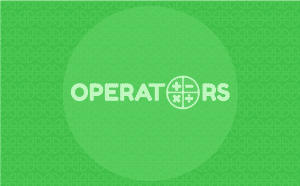# OperatorsExtension Description
Operator blocks deal with many mathematical functions within a project.

## Introduction

Operators is one of the ten categories of default Scratch blocks. They are color-coded light-green and are used to script math equations and string handling.

## PictoBlox Blocks

The block checks if the first value is less than the second value. If it is less, the block returns true; if not, it returns false. This block works with letters too, as well as numbers. In Scratch, letters at the top of the alphabet (e.g. a, b, c) are worth less than letters at the end (e.g. x, y, z).
The block checks if the first value is equal to the other value. If the values are equal, the block returns true; if not, false. This block is not case-sensitive.
The block checks if the first value is greater than the other value. If the second value is less, the block returns true; if not, it returns false.
The block joins two Boolean blocks so they both have to be true to return true. If they are both true, the block returns true; if they are not all true or none true, it returns false.
The block joins two Boolean blocks so any one of them can be true to return true — if at least one of them is true, the block returns true; if neither of them are true, it returns false.
The block checks if the Boolean inside it is false — if it is false, the block returns true; if the condition is true, it returns false.
The block adds two values and reports the result. The numbers can be typed directly into the block, or Reporter blocks can be used instead. This block can be stacked inside itself; this can be used to fit more numbers in.
The block subtracts the second value from the first and reports the result.
The block multiplies the two values and reports the result.
The block divides the second value from the first and returns the result. If the first value is not evenly divisible by the second, the reported value will have decimals. To find the remainder instead, use the () Mod () block. Note, dividing by 0 in the online editor will return infinity, 0, or negative infinity. It depends on if the numerator is positive, 0, or negative; respectively.
The block picks a pseudorandom number ranging from the first given number to the second, including both endpoints. If both numbers have no decimals, it will report a whole number. For example, if a 1 and a 3 were inputted, the block could return a 1, 2 or 3. If one of the numbers has a decimal point, even .0, it reports a number with a decimal. For example, if 0.1 and 0.14 were given, the output will be 0.1, 0.11, 0.12, 0.13, or 0.14.
The block concatenates, or “links” the two values together and reports the result — for example, if “hello” and “world” were put in the block, it would report “helloworld”.
The block reports the specified characteristics of the given text. Even though the block says “letter”, it will report all characters, including letters, numbers, symbols, and even spaces.
The block reports how many characters the given string contains.
The block reports the remainder of the division when the first value is divided by the second. For example, when 10 is put in the first input and 3 in the second, the block will report 1; 10 divided by 3 gives a remainder of 1.
The block rounds the given number to the nearest integer. It follows the standard rules of rounding; decimals that are .5 or higher are rounded up, whereas decimals less than .5 are rounded down.
The block performs a specified function on a given number and reports the result. The function can be changed by clicking the down arrow and selecting a new function from the drop-down menu.